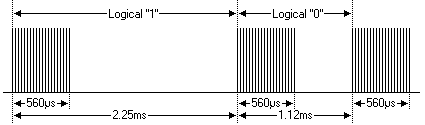## 2014年12月12日 星期五

### LIRC 紅外線解碼程式解說NEC Infrared Protocol

• A 9ms leading pulse burst (16 times the pulse burst length used for a logical data bit)
• A 4.5ms space
• The 8-bit address for the receiving device
• The 8-bit logical inverse of the address
• The 8-bit command
• The 8-bit logical inverse of the command
• Final 562.5µs pulse burst to show end of message transmission.
• Logical '0' – a 562.5µs pulse burst followed by a 562.5µs space, with a total transmit time of 1.125ms
• Logical '1' – a 562.5µs pulse burst followed by a 1.6875ms space, with a total transmit time of 2.25

Pulse 係由很多 on/off 交替的訊號所組成:
• Carrier frequency = 38kHz
• duty factor = 1/3
• period = 26.5us16 bits for the address (address + inverse) require 27ms to transmit time .and the 16 bits for the command (command + inverse) also require 27ms to transmit time.
because (address + address inverse) or (command+command inverse) will always contain 8 '0's  and 8 '1's    so (8 * 1.125ms) + (8 * 2.25ms) == 27 ms .
according to this total time required to transmit the frame is (9ms +4.5ms +27ms+27ms) = 67.5 ms.

If the key on the remote controller is kept depressed, a repeat code will be issued, typically around 40ms after the pulse burst that signified the end of the message. A repeat code will continue to be sent out at 108ms intervals, until the key is finally released. The repeat code consists of the following, in order:

if user keeps the key depressed the repeat codes keep coming

==========
static irqreturn_t cir_irq_handler (int irq, void *dev_id, struct pt_regs *regs)
{
struct timeval tv;
long deltv;
int data;
struct cir_device_data *dev;

dev = (struct cir_device_data *)dev_id;

/* get current time */
do_gettimeofday(&tv);

if(dev->ir_state == IR_STATE_0) {
dev->ir_state = IR_STATE_1;
/* Initializate ir variable */
dev->ir_count = 0;
dev->complete_bits = 0;

/* Configure to generate an interrupt on rising edge
* Wait 9ms pre-pulse.
*/
set_irq_type(gpio_to_irq(CIR_GPIO), IRQT_RISING);
} else {
deltv = tv.tv_sec - dev->lasttv.tv_sec;

/* calc time since last interrupt in microseconds */
data = (int) (deltv*1000000 + tv.tv_usec - dev->lasttv.tv_usec);
decode_process(dev, data);
}

/* restore time */
dev->lasttv = tv;

return IRQ_HANDLED;
}

static int check_bit(int interval)
{
int bit = 0;

/* if interval is 1.12ms(+-20%) then bit 0 */
if(interval > 896 && interval < 1344)
bit = 0;
/* if interval is 2.25ms(+-20%) then bit 1 */
else if(interval > 1800 && interval < 2700)
bit = 1;
else
/* invalid data bit */
bit = -1;

return bit;
}

static void decode_process(struct cir_device_data *dev, int interval)
{
int bitvalue = 0;

switch(dev->ir_state){
case IR_STATE_1:
/* Configure to generate an interrupt on falling edge
* decision 9ms pre-pulse, within a +-20% range of the nominal value
* 9000 - (9000*0.2) = 7200, 9000+(9000*0.2) = 9900
*/
set_irq_type(gpio_to_irq(CIR_GPIO), IRQT_FALLING);
if(interval > 7200 && interval < 10800)
dev->ir_state = IR_STATE_2; // pre-pulse detected.
else
dev->ir_state = IR_STATE_0; // exit with error, return state_0
break;
case IR_STATE_2:
/* decision long interval(4.5ms) or short interval (2.25ms)
* long interval is normal message, short interval is repetition code.
* 4500 - (4500*0.2) = 3600, 4500 + (4500*0.2) = 5400
* 2250 - (2250*0.2) = 1800, 2250 + (2250*0.2) = 2700
*/
if(interval > 3600 && interval < 5400) {
dev->ir_state = IR_STATE_3;
} else if(interval > 1800 && interval < 2700) {
/* driver not supported repetition.
* Return state_0
*/
dev->ir_state = IR_STATE_0; //repeat state.
} else {
dev->ir_state = IR_STATE_0;     // exit with error, return state_0
printk(KERN_DEBUG "CIR:exit with error!\n");
}
break;
case IR_STATE_3:
/* 32 bits entire message receiving
*/
bitvalue = check_bit(interval);
if(bitvalue < 0) {
/* invalid data bit */
dev->ir_state = IR_STATE_0;
} else {
dev->complete_bits <<= 1;
dev->complete_bits |= bitvalue;
dev->ir_state = IR_STATE_3;
dev->ir_count++;

if(dev->ir_count >= CIR_DATA_BITS) {
/* receiving complete */
dev->ir_state = IR_STATE_0;
/* store to buffer */
wake_up_interruptible(&dev->waitq);
}
}
break;
default:
break;
}
}